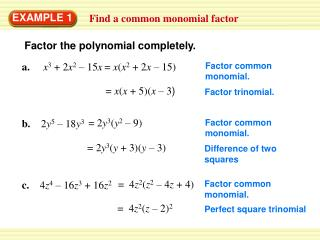DownloadDownload PresentationEXAMPLE 1

# EXAMPLE 1

Download Presentation## EXAMPLE 1

- - - - - - - - - - - - - - - - - - - - - - - - - - - E N D - - - - - - - - - - - - - - - - - - - - - - - - - - -
##### Presentation Transcript

1. EXAMPLE 1 Find a common monomial factor Factor the polynomial completely. a. x3 + 2x2 – 15x = x(x2 + 2x – 15) Factor common monomial. = x(x + 5)(x – 3) Factor trinomial. = 2y3(y2 – 9) b. 2y5 – 18y3 Factor common monomial. = 2y3(y + 3)(y – 3) Difference of two squares = 4z2(z2 – 4z + 4) c. 4z4 – 16z3 + 16z2 Factor common monomial. = 4z2(z – 2)2 Perfect square trinomial

2. = 2z2 (2z)3 – 53 EXAMPLE 2 Factor the sum or difference of two cubes Factor the polynomial completely. = x3 + 43 a. x3 + 64 Sum of two cubes = (x + 4)(x2 – 4x + 16) = 2z2(8z3 – 125) b. 16z5 – 250z2 Factor common monomial. Difference of two cubes = 2z2(2z – 5)(4z2 + 10z + 25)

3. for Examples 1 and 2 GUIDED PRACTICE Factor the polynomial completely. 1. x3 – 7x2 + 10x SOLUTION x3 – 7x2 + 10x = x3 – 7x2 + 10x = x( x2 – 7x + 10) Factor common monomial. = x( x – 5 )( x – 2 ) Factor trinomial.

4. for Examples 1 and 2 GUIDED PRACTICE 2. 3y5 – 75y3 SOLUTION 3y5 – 75y3 = 3y3 (y2 – 25) Factor common monomial. = 3y3 (y – 5)( y + 5 ) Difference of two squares

5. for Examples 1 and 2 GUIDED PRACTICE 3. 16b5 + 686b2 SOLUTION 16b5 + 686b2 = 2b2 (8b3 + 343) Factor common monomial. = 2b2 (2b + 7)(4b2 –14b + 49 ) Difference of two cubes

6. for Examples 1 and 2 GUIDED PRACTICE 4. w3 – 27 SOLUTION w3 – 27 =w3 – (3)3 =(w – 3)(w2 + 3w + 9) Difference of two cubes